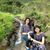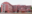Trending ▼   ResFinder# ICSE Board Exam 2018 : English Paper 1 (English Language)FIRST!!!

8 pages, 47 questions, 44 questions with responses, 179 total responses,40Subham Mishra Ryan International School (RIS) ICSE, Kharghar, Navi Mumbai
+Fave Message
 Home > subham101 >   F Also featured on: icseFormatting page ...

E N G L IS H L A IV G U A G E E N G L IS H P a p e r (T w A m vers Yo to th is P ill n u w e g iv e n t be o T h is tim t h e tim llo a w e is to b e e he a d a t th e be c p e r m its t d to Yo te n ded m a r ( r e a a v is e u l s fo d to sp e n a n Qu (D e s t io n d a (a ) W te A fo o re N m Y an o u n ey d 20 400 in m u qu u r p a r ts o es e a l o w tio n s in m te s in a n s w e r in g u d e in m se pa ra e y u te s paper jo r a re te s in id e d ]5 e s tio n q u e s tio n s 35 v g iv w r itin g th e a n s w e rs in b r a c ke ts en a n s w e r in g Qu e s tio n [] 1 2 Q u e s tio n l E x p la in ha d be en w t fr o m ha t yo w a itin g ds y o fe e lin g s ? D e s c r ib e in a tio n s ta r te h a p p in r y an title d en u u yo ' o n e o f th e fo llo w [2 5 ] in g : G / e E x p re s s y o " ess d id an o u ts id e he a rd ho w u r ow n w d w ex p e r ie n u r v ie w ce w s e ith e r fo r o r h e n y o u h e lp e d to p r e p a r e h a t y o u g a in e d f o m th e th e e x a m he n yo u th e s c e n e c h a n in a tio n h a ll D a iT iv e ge d d a o n ce ex e s c r ib e w t th e p la c e yo u p e r ie n e n te r e ce hat yo W Dat a u w e re saw yo u r d th e h a ll a n d th e d T h is P a p e r T 18 0 1 1 )o n ) s ta te m e n t in c id e n so u n w o rd s l s h o r t s to r y is im p o r a n fo e an d th e ex am li o p ro Jir s t d in g th e q u th a n 3 5 m in u te s o n tM s q u e s tio n a i o r ig in a g a in s t th is m ea (d ) m c o m p o o n (3 5 0 W te (c ) a t m o r e th a n n o th e p a p e r 1 o n o t sp e n a d o n ite d u r in g th e w r e s tio n s o r qu r ) P a p e r is th e tim A tte m p t r h e in itte n w r u rs s p e n t in r e a f th is o ho o 1 c o n s is ts o f 8 p r in te d p a g e s T u m O v e r ? C o p y r ig h t R e s e r v e d Scanned by CamScannerFormatting page ...

Top Contributors
to this ResPaperRhea Thomas(29)Saaksshi Singh(20)Pavan Sd(19)Charul Jain(18)Formatting page ...Formatting page ...Formatting page ...Formatting page ...Formatting page ...Formatting page ...